# C Program to implement Bubble sort Algorithm

In this tutorial you will learn about the C Program to implement Bubble sort Algorithm and its application with practical example.

## C Program to implement Bubble sort Algorithm

In this tutorial, we will learn to create a C program that will do Bubble sort using C programming.

## Prerequisites

Before starting with this tutorial we assume that you are best aware of the following C programming topics:

• Operators in C Programming.
• Basic Input and Output function in C Programming.
• Basic C programming.
• For loop in C Programming.
• Creating and Using the user-defined function in C programming.

## What is Bubble Sort?

In every programming language, the sorting of data is a very important factor.  In C Language. Many different techniques for sorting are available to work with but in this tutorial, we will focus on the Bubble Sort Technique.

In this technique, the elements of the array are sorted from the very first value by swapping from starts first & second and going to till the end. Now, this process will go on until the array is completely arranged.

## Output :-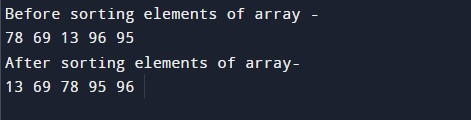In the above program, we have first declared and initialized a set variables required in the program.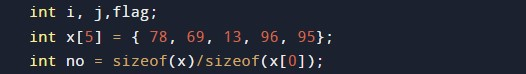• no = it will hold the size of the array.
• x= it will hold the elements in an array.
• flag= it will hold the temporary values.
• i= it will hold the integer value to control the array.
• j= it will hold the integer value to control the array.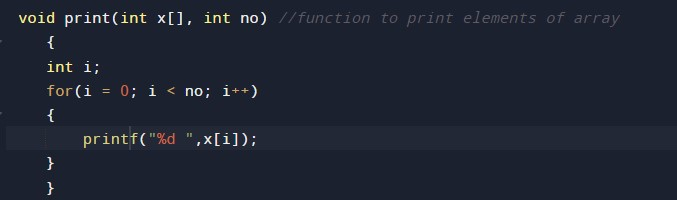Function to print on the terminal/console.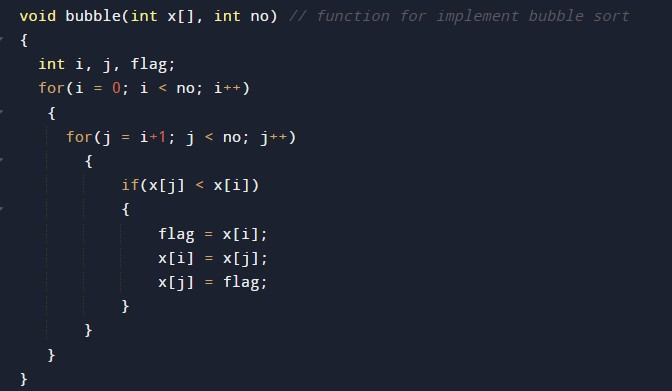Function to implement the bubble sort.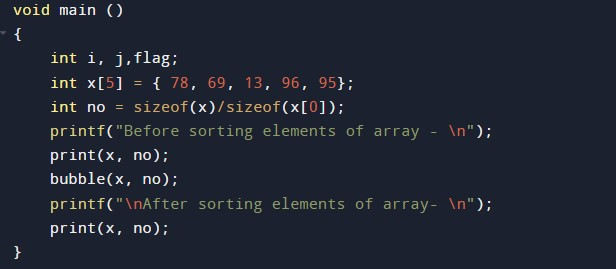The main function to perform and navigate the program cleanly.

In this tutorial we have learn about the C Program to implement Bubble sort Algorithm and its application with practical example. I hope you will like this tutorial.